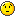# TTree possible with variable-length std::vectors?

Hi ROOTers,

I’d like to construct a ROOT TTree that can store multiple, different sized std::vectors in a single event stored in the TTree. (I am aware that ROOT tutorial tree3.C shows how to implement variable-size arrays, but for external reasons std::vectors would be very preferable.) The application is to store 8 digitized waveforms of variable length from a single trigger, therefore, I would like to have a TTree that can store 8 std::vectors of size containing the waveforms in a single TTree entry.

I’ve written a very simple example based on the tree3.C tutorial that stores two variable-sized vectors on separate branches for the same entries. Interestingly, the code seem to work perfectly from within ROOT using the CINT interpreter. However, when compiled using GCC and run as a standalone executable, a seg fault occurs when trying to read from the TTree object. For my application, the code must run as part of a standalone executable so I’d like to resolve this issue.

Questions:

1. Is it possible to store variable-sized std::vectors in a TTree entry as I am attempting?
2. Why does the attached code work from CINT but not when compiled as a standalone executable?

Thanks much for an insight on this issue.

~Zach

``````#include "TFile.h"
#include "TTree.h"
#include "TRandom.h"

#include <vector>
#include <iostream>
using namespace std;

void WriteTree()
{
TTree *T = new TTree("T","T");
TFile F("T.root","recreate");

vector<int> Vec1;
vector<int> Vec2;

T->Branch("Vec1", &Vec1);
T->Branch("Vec2", &Vec2);

const int NumEvents = 5;

const int MaxLength = 10;

for(int i=0;i<NumEvents;i++) {

Vec1.clear();
Vec2.clear();

int RandomLength1 = gRandom->Rndm()*(MaxLength-1);
int RandomLength2 = gRandom->Rndm()*(MaxLength-1);

for(int n=0; n<RandomLength1; n++)
Vec1.push_back(n*2);

for(int n=0; n<RandomLength2; n++)
Vec2.push_back(n*3);

cout << "Size of Vec1 (write) = " << Vec1.size() << "\n"
<< "Size of Vec2 (write) = " << Vec2.size() << "\n"
<< endl;

T->Fill();
}
T->Write();
}

{
TFile *F = new TFile("T.root");
TTree *T = (TTree*)F->Get("T");

int Arr;
vector<int> *Vec1, *Vec2;

for(int evt=0; evt<T->GetEntries(); evt++){
T->GetEntry(evt);

size_t Length1 = (*Vec1).size();
size_t Length2 = (*Vec2).size();

cout << "Size of Vec1 = " << Length1 << endl;
for(int i=0; i<Length1; i++)
cout << "Vec1[" << i << "] = " << (*Vec1)[i] << endl;

cout << "Size of Vec2 = " << Length2 << endl;
for(int i=0; i<Length2; i++)
cout << "Vec2[" << i << "] = " << (*Vec2)[i] << endl;

cout << "\n" << endl;
}
}

int TreeTest()
{
WriteTree();

return 0;
}
``````

Problem fix … in the “ReadTree” routine use: `vector<int> *Vec1 = 0, *Vec2 = 0;`
Advice … in the end of the “ReadTree” routine add: `T->ResetBranchAddresses();`
Search also for “GetObject” in http://root.cern.ch/root/html/TDirectoryFile.html#TDirectoryFile:Get and/or http://root.cern.ch/root/html/TDirectory.html#TDirectory:Get

Dear Pepe,:: sigh ::

I suppose everyone get’s his/her chance for an embarrassing post to RootTalk. It had been a long day, and it looks like I overlooked that simple initialization. Thanks very much for pointing this out; code seems to work perfectly now.

~Zach